Courses

# Thermodynamic Equation Of State - Thermodynamic Chemistry Notes | EduRev

## Physical Chemistry

Created by: Asf Institute

## Chemistry : Thermodynamic Equation Of State - Thermodynamic Chemistry Notes | EduRev

The document Thermodynamic Equation Of State - Thermodynamic Chemistry Notes | EduRev is a part of the Chemistry Course Physical Chemistry.
All you need of Chemistry at this link: Chemistry

Thermodynamic equation of state:

(1)   First thermodynamic equation of state: The charge in internal energy with respect to volume at constant temperature is known as first thermodynamic equation of state. i.e.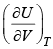Dimensionally it is equal to pressure.  Thus it is also called internal pressure (π).
We know that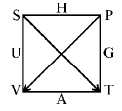dU = TdS – PdV

∴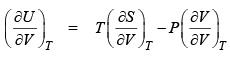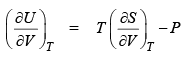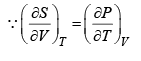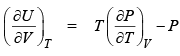(2)  Second thermodynamic equation of state: The change in enthalpy w.r.t. pressure at constant temperature is known as second thermodynamic equation of state.

dH = TdS + VdP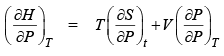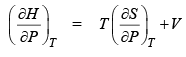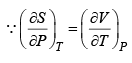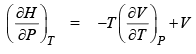Comparison of Isothermal and Adiabatic Expansions: Let us consider isothermal and adiabatic expansio ns of an ideal gas from initial volume Vi and pressure Pi to a commo n final vo lume Vf.  If Piso and Padia are final pressure, then

PiVi = Piso Vf                               (for isothermal expansio n)
and PiVi r = Padia Vf r                (for adiabat ic expansio n)
Accordingly,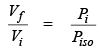and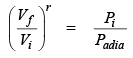Since for expansio n Vf > Vi and for all r > 1, hence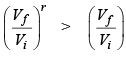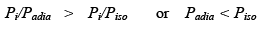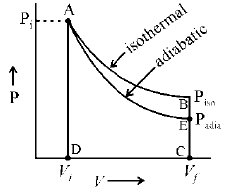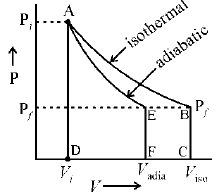From graph (a) & (b), it is clear that work done in isothermal expansion (shown by area ABCD) is greater than the work done in adiabatic expansion (shown by area AECD).
Consider the expansions in which the final pressure Pf is the same in both cases.  If Viso and Vadia are the final volume is isothermal and adiabatic expansion then

PiVi = Pf Viso                        (for isothermal expansion)
then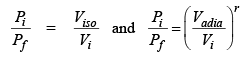⇒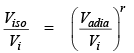or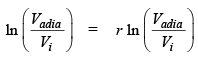[∵ r > 1 then]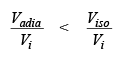or     Vadia < Viso

Reversible Isothermal expansion of a Real gas Using vendor walls equation we find the expression for W, ΔV, ΔH and q for reversible isothermal expansion of real gas.

Work of expansion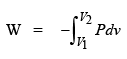For the vendor walls gas,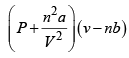= nRT so that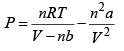Hence,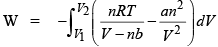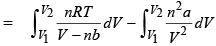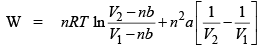Internal energy change

We know that,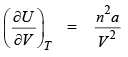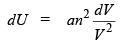(at constant temperature)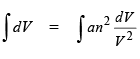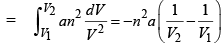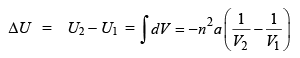Enthalpy change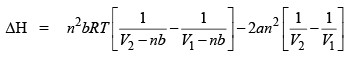Heat change. We know that ΔU = q + w or q = ΔU - w
Subst ituting the value of w & ΔU in this equation we get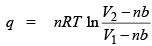Comparison of Work of Expansion of an ideal gas and a real gas.
We know that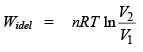and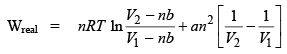If  V >> nb, than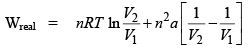Hence,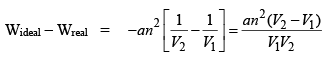Since for the expansio n of a gas, V2 > V1 then
Wideal > Wreal

Offer running on EduRev: Apply code STAYHOME200 to get INR 200 off on our premium plan EduRev Infinity!

## Physical Chemistry

77 videos|83 docs|32 tests

,

,

,

,

,

,

,

,

,

,

,

,

,

,

,

,

,

,

,

,

,

;